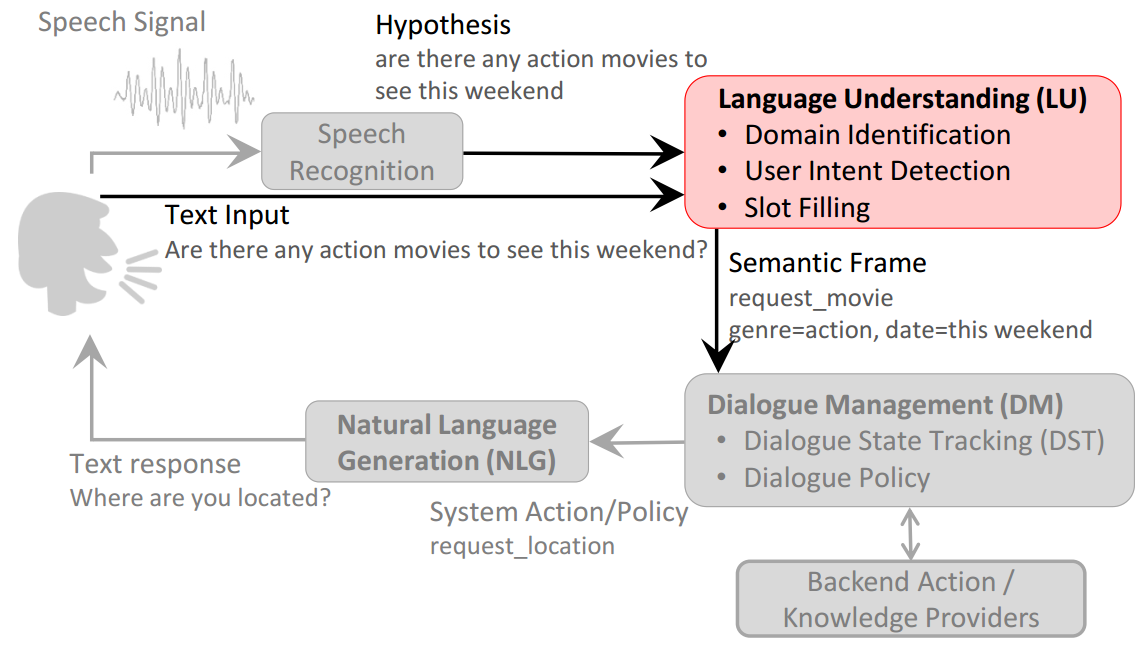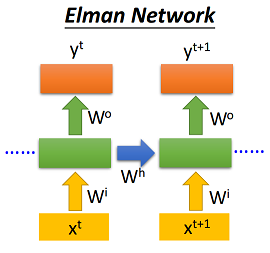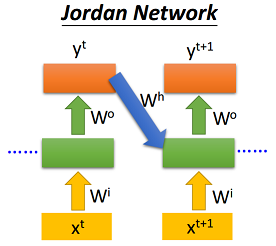# 1. 概况

## 1.1 任务SLU主要通过如下三个子任务来理解用户的语言：

1. 领域识别(Domain Detection)
2. 用户意图检测(User Intent Determination)
3. 语义槽填充(Semantic Slot Filling)

## 1.2 数据集

what	O
is	O
the	O
arrival	B-flight_time
time	I-flight_time
in	O
san	B-fromloc.city_name
francisco	I-fromloc.city_name
for	O
the	O
DIGITDIGITDIGIT	B-depart_time.time
am	I-depart_time.time
flight	O
leaving	O
washington	B-fromloc.city_name


ATIS数据集一共有83种语义槽，因此序列标注的标签类别一共有$83+83+1=167$个。ATIS数据集分为训练集和测试集，数据规模如下表：

# 2. 模型

RNN可以分为简单RNN(Simple RNN)和门控机制RNN(Gated RNN)，前者的RNN单元完全接收上个时刻的输入；后者基于门控机制，通过学习到的参数自行决定上个时刻的输入量和当前状态的保留量。下面将介绍Elman-RNN, Jordan-RNN, Hybrid-RNN(Elman和Jordan结合)这三种简单RNN，以及经典的门控机制RNN：LSTM。

## 2.1 Elman-RNN

Elman-RNN将当前时刻的输入$x_t$和上个时刻的隐状态输出$h_{(t-1)}$作为输入，具体如下：

$\begin{split}\begin{array}{ll}h_t = \sigma(W_{ih} x_t + b_{ih} + W_{hh} h_{(t-1)} + b_{hh}) \end{array}\end{split}$class ElmanRNNCell(nn.Module):
def __init__(self, input_size, hidden_size):
super(ElmanRNNCell, self).__init__()
self.hidden_size = hidden_size
self.i2h_fc1 = nn.Linear(input_size, hidden_size)
self.i2h_fc2 = nn.Linear(hidden_size, hidden_size)
self.h2o_fc = nn.Linear(hidden_size, hidden_size)

def forward(self, input, hidden):
hidden = F.sigmoid(self.i2h_fc1(input) + self.i2h_fc2(hidden))
output = F.sigmoid(self.h2o_fc(hidden))
return output, hidden


## 2.2 Jordan-RNN

Jordan-RNN将当前时刻的输入$x_t$和上个时刻的输出层输出$y_{(t-1)}$作为输入，具体如下：

$\begin{split}\begin{array}{ll}h_t = \sigma(W_{ih} x_t + b_{ih} + W_{yh} y_{(t-1)} + b_{yh}) \end{array}\end{split}$class JordanRNNCell(nn.Module):
def __init__(self, input_size, hidden_size):
super(JordanRNNCell, self).__init__()
self.hidden_size = hidden_size
self.i2h_fc1 = nn.Linear(input_size, hidden_size)
self.i2h_fc2 = nn.Linear(hidden_size, hidden_size)
self.h2o_fc = nn.Linear(hidden_size, hidden_size)

def forward(self, input, hidden=None):
if hidden is None:
hidden = self.y_0
hidden = F.sigmoid(self.i2h_fc1(input) + self.i2h_fc2(hidden))
output = F.sigmoid(self.h2o_fc(hidden))
return output, output


## 2.4 Hybrid-RNN

Hybrid-RNN将当前时刻的输入$x_t​$，上个时刻的隐状态$h_{(t-1)}​$ 以及上个时刻输出层输出$y_{(t-1)}​$作为输入，具体如下：

$\begin{split}\begin{array}{ll}h_t = \sigma(W_{ih} x_t + b_{ih} + W_{hh} h_{(t-1)} + b_{hh} + W_{yh} y_{(t-1)} + b_{yh}) \end{array}\end{split} ，并且y_0初始化为可训练的参数。使用Pytorch具体实现如下: python class HybridRNNCell(nn.Module): def __init__(self, input_size, hidden_size): super(HybridRNNCell, self).__init__() self.hidden_size = hidden_size self.i2h_fc1 = nn.Linear(input_size, hidden_size) self.i2h_fc2 = nn.Linear(hidden_size, hidden_size) self.i2h_fc3 = nn.Linear(hidden_size, hidden_size) self.h2o_fc = nn.Linear(hidden_size, hidden_size) self.y_0 = nn.Parameter(nn.init.xavier_uniform(torch.Tensor(1, hidden_size)), requires_grad=True) def forward(self, input, hidden, output=None): if output is None: output = self.y_0 hidden = F.sigmoid(self.i2h_fc1(input)+self.i2h_fc2(hidden)+self.i2h_fc3(output)) output = F.sigmoid(self.h2o_fc(hidden)) return output, hidden  ## 2.5 LSTM LSTM引入了**记忆单元**c_t和3种控制门，包括**输入门**(input gate)i_t，**遗忘门**(forget gate)f_t，**输出门**(output gate)o_t， 首先，输入层接受当前时刻输入x_t和上个时刻隐状态输出h_{(t-1)}，通过\tanh激活函数得到记忆单元的输入g_t； 然后遗忘门f_t决定上个时刻记忆单元c_{(t-1)}的保留比例，输入门i_t决定当前时刻记忆单元的输入g_t的保留比例，两者相加得到当前的记忆单元c_t； 最后记忆单元c_t通过\tanh激活函数得到的值在输出门o_t的控制下得到最终的当前时刻隐状态h_t， 具体如下： \begin{split}\begin{array}{ll}i_t = \sigma(W_{ii} x_t + b_{ii} + W_{hi} h_{(t-1)} + b_{hi}) \\f_t = \sigma(W_{if} x_t + b_{if} + W_{hf} h_{(t-1)} + b_{hf}) \\g_t = \tanh(W_{ig} x_t + b_{ig} + W_{hg} h_{(t-1)} + b_{hg}) \\o_t = \sigma(W_{io} x_t + b_{io} + W_{ho} h_{(t-1)} + b_{ho}) \\c_t = f_t c_{(t-1)} + i_t g_t \\h_t = o_t \tanh(c_t)\end{array}\end{split}$

Pytorch已经实现了LSTM, 只需要调用相应的API即可，调用的代码片段如下:

self.rnn = nn.LSTM(input_size=embedding_dim,
hidden_size=hidden_size,
bidirectional=bidirectional,
batch_first=True)


# 3. 实验

## 3.1 实验设置

• 所有RNN模型均只使用单层；
• 词向量维度设置为100维，并且随机初始化，在训练过程中进行调整；
• 隐状态维度设置为75维;
• 采用带动量的随机梯度下降(SGD)，batch size为1，学习率(learning rate)为0.1，动量(momentum)为0.9并保持不变;
• epoch=10；
• 每种RNN模型都实现单向(Single)和双向(Bi-Directional)，并分别训练。

## 3.2 实验结果

$F_1(\%) / T(s)$ Elman Jordan Hybrid LSTM
Single 87.26 / 438 87.90 / 487 88.46 / 494 92.16 / 3721
Bi-Directional 92.88 / 565 90.31 / 580 91.85 / 613 93.75 / 4357

• 基于门控机制的LSTM由于其参数和运算步骤的增加，一个epoch的训练时长是另外三种Simple RNN的9倍左右，而$F_1$得分也比Simple RNN高；
• 双向(Bi-Directional)RNN的$F_1$得分普遍比单向(Single)RNN高，而运行时间也多一些。

$F_1(\%) / T(s)$ Elman Jordan Hybrid LSTM
Single 88.89 / 35.2 88.36 / 41.3 89.65 / 43.5 92.44 / 16.8
Bi-Directional 91.78 / 68.0 89.82 / 72.2 93.61 / 81.6 94.26 / 18.7

# 4. 总结与展望

• 改变词向量维度和隐状态维度；
• 考虑采用预训练词向量，然后固定或者进行微调；
• 采用正则化技术，包括L1/L2, Dropout, Batch Normalization, Layer Normalization等；
• 增加epoch次数。

# 参考资料

Mesnil G, Dauphin Y, Yao K, et al. Using recurrent neural networks for slot filling in spoken language understanding[J]. IEEE/ACM Transactions on Audio, Speech, and Language Processing, 2015, 23(3): 530-539.

Wikipedia. Recurrent neural network. https://en.wikipedia.org/wiki/Recurrent_neural_network

PyTorch documentation. Recurrent layers. http://pytorch.org/docs/stable/nn.html#recurrent-layers

Hung-yi Lee. Machine Learning (2017,Spring). http://speech.ee.ntu.edu.tw/~tlkagk/courses/ML_2017/Lecture/RNN.pdf

YUN-NUNG (VIVIAN) CHEN. Spring 105 - Intelligent Conversational Bot. https://www.csie.ntu.edu.tw/~yvchen/s105-icb/doc/170321_LU.pdf

posted @ 2018-05-01 23:26  llhthinker  阅读(7044)  评论(1编辑  收藏
TOP